### Home > PC3 > Chapter 7 > Lesson 7.1.4 > Problem7-54

7-54.

The graphs of $y=f(x)$, $y=g(x)$, and $y=h(x)$, are shown below. Far below are the graphs of the reciprocal of two of the functions from above. Express $a(x)$ and $b(x)$ in terms of one of the functions listed above.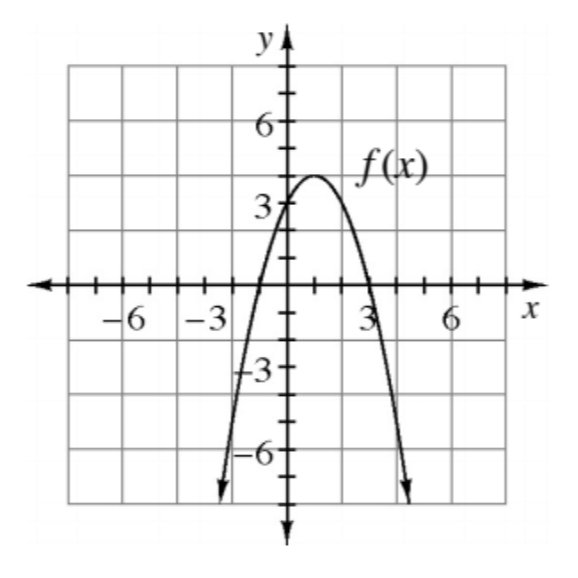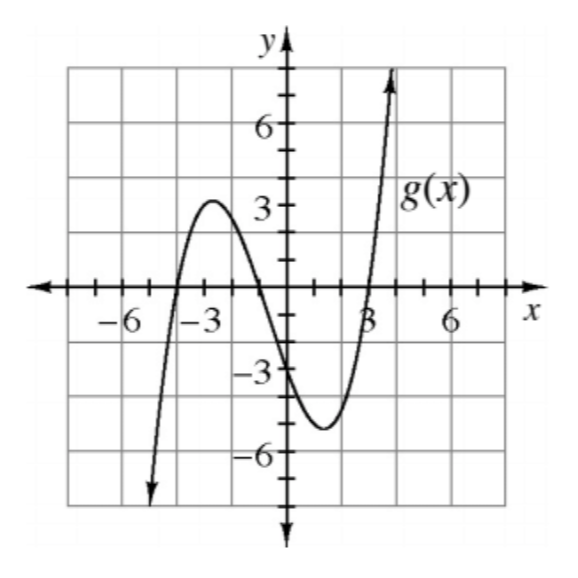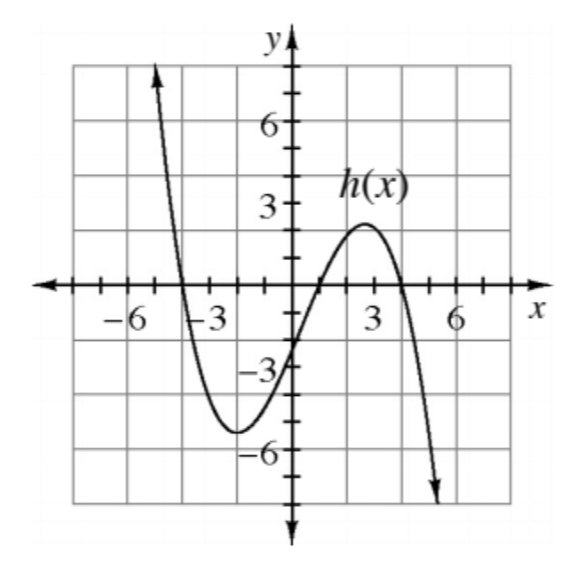When $f(x)=0$, then $\frac{1}{f\left(x\right)}$ is undefined, creating a vertical asymptote.

1.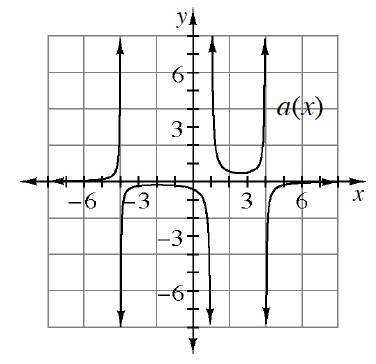1.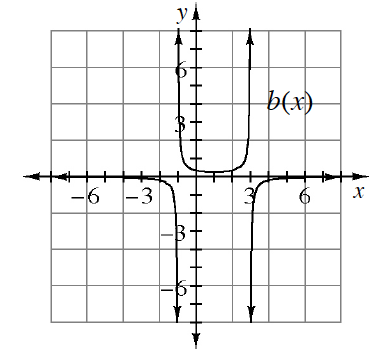1. Sketch the reciprocal of the function that was not used in parts (a) or (b).